Win up to 100% scholarship on Aakash BYJU'S JEE/NEET courses with ABNAT Win up to 100% scholarship on Aakash BYJU'S JEE/NEET courses with ABNAT

# JEE Main 2022 June 29 – Shift 2 Chemistry Question Paper with Solutions

The JEE Main 2022 June 29 Shift 2 Chemistry question paper with solutions are provided here to assist students in obtaining the right answers. They can also access solutions to all the JEE main 2022 question papers, which have been solved by our subject experts. Practising multiple JEE main question papers will improve your skills and understanding of the exam pattern, and familiarise you with the various types of questions asked in the exams. This way of preparation will improve your scores in the upcoming JEE session. Below are the detailed solutions for the June 29 second shift chemistry JEE main 2022 question paper.

## JEE Main June 29th 2022 Shift 2 Chemistry Paper and Solutions

#### SECTION – A

Multiple Choice Questions: This section contains 20 multiple choice questions. Each question has 4 choices (1), (2), (3) and (4), out of which ONLY ONE is correct.

1. Using the rules for significant figures, the correct answer for the expression

$$\begin{array}{l}\frac{0.02858 \times 0.112}{0.5702}\end{array}$$
will be

(A) 0.005613

(B) 0.00561

(C) 0.0056

(D) 0.006

Sol.

$$\begin{array}{l}\frac{0.02858 \times 0.112}{0.5702} = .00561\end{array}$$

The answer is expressed in 3 significant figures.

2. Which of the following is the correct plot for the probability density ψ2(r) as a function of distance ‘r’ of the electron from the nucleus for 2s orbital?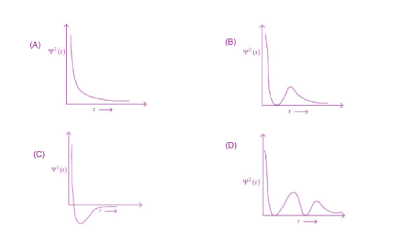Sol. 2s

Radial node = n – l – 1

= 2 – 0 – 1

= 1

It will have one radial node.

3. Consider the species

$$\begin{array}{l}\textup{CH}_4, \textup{NH}_4^+\ \text{and}\ \textup{BH}_4^- .\end{array}$$
Choose the correct option with respect to these species.

(A) They are isoelectronic and only two have tetrahedral structures

(B) They are isoelectronic and all have tetrahedral structures.

(C) Only two are isoelectronic and all have tetrahedral structures.

(D) Only two are isoelectronic and only two have tetrahedral structures.

Sol.

$$\begin{array}{l}\text{CH}_4,\text{NH}_4^+~ \text{and}~ \text{BH}_4^-\end{array}$$
are isoelectronic as well as tetrahedral.

4. 4.0 moles of argon and 5.0 moles of PCl5 are introduced into an evacuated flask of 100 litre capacity at 610 K. The system is allowed to equilibrate. At equilibrium, the total pressure of mixture was found to be 6.0 atm. The Kp for the reaction is [Given : R = 0.082 L atm K–1 mol–1]

(A) 2.25

(B) 6.24

(C) 12.13

(D) 15.24

Sol.

$$\begin{array}{l}\text{PCl}_5 \rightleftharpoons \text{PCl}_3 + \text{Cl}_2\end{array}$$

t = 0 5 0 0

t = t 5 – n n n

Total moles = 5 – n + n + n

= 5 + n.

For Argon

nAr = 4

Total moles = nAr + nPCl5 + nPCl3 + nPCl2

= 4 + 5 + n

= 9 + n

$$\begin{array}{l}K_p =\frac{\mathrm{P}_{\mathrm{PCl}_{3}} \cdot \mathrm{PCl}_{2}}{\mathrm{P}_{\mathrm{PCl}_{5}}}\end{array}$$

PV = nRT

6 × 100 = (9 + n) × 0.082 × 610

n = 3

\begin{array}{l}\begin{aligned}&=\frac{\left(\frac{3}{12} \times 6\right) \times\left(\frac{3}{12} \times 6\right)}{\frac{2}{12} \times 6} \\&=\frac{27}{12}=\frac{9}{4}=2.25\ atm\end{aligned}\end{array}

5. A 42.12% (w, v) solution of NaCl causes precipitation of a certain sol in 10 hours. The coagulating value of NaCl for the sol is

[Given : Molar mass : Na = 23.0 g mol–1; Cl = 35.5 g mol–1]

(A) 36 mmol L–1

(B) 36 mol L–1

(C) 1440 mol L–1

(D) 1440 mmol L–1

Sol. Data is insufficient.

6. Given below are two statements. One is labelled as Assertion A and the other is labelled as Reason R.

Assertion A: The first ionization enthalpy for oxygen is lower than that of nitrogen.

Reason R: The four electrons in 2p orbitals of oxygen experience more electron-electron repulsion.

In the light of the above statements, choose the correct answer from the options given below.

(A) Both A and R are correct and Rj is the correct explanation of A

(B) Both A and R are correct but R is NOT the correct explanation of A

(C) A is correct but R is not correct

(D) A is not correct but R is correct

Sol. Nitrogen has half filled p-orbitals which is stable. Due to this it’s 1st ionization energy is more than oxygen.

7. Match List-I with List-II

 List-I Ore List-II Composition A. Siderite I. FeCO3 B. Malachite II. CuCO3. Cu(OH)2 C. Sphalerite III. ZnS D. Calamine IV. ZnCO3

Choose the correct answer from the options given below:

(A) A-I, B-II, C-III, D-IV (B) A-III, B-IV, C-II, D-I

(C) A-IV, B-III, C-I, D-II (D) A-I, B-II, C-IV, D-III

Sol. Siderite → FeCO3

Malachite → CuCO3. Cu(OH)2

Sphalerite → ZnS

Calamine → ZnCO3

8. Given below are two statements.

Statement-I: In CuSO4.5H2O, Cu-O bonds are present.

Statement-II: In CuSO4.5H2O, ligands coordinating with Cu(II) ion are O-and S-based ligands.

In the light of the above statements, choose the correct answer from the options given below:

(A) Both Statement-I and Statement-II are correct

(B) Both Statement-I and Statement-II are incorrect

(C) Statement-I is correct but Statement-II is incorrect

(D) Statement-I is incorrect but Statement-II is correct.

Sol. Statement I is true but statement II is false. Only oxygen atom forms Co-ordinate bond with Cu+2 in CuSO4.5H2O

9. Amongst baking soda, caustic soda and washing soda, carbonate anion is present in

(A) Washing soda only

(B) Washing soda and caustic soda only

(C) Washing soda and baking soda only

(D) Baking soda, caustic soda and washing soda

Sol.

$$\begin{array}{l}\text{CO}_3^{2-}\end{array}$$
ion is present only in washing soda.

10. Number of lone pair(s) of electrons on central atom and the shape of BrF3 molecule respectively, are

(A) 0, triangular planar

(B) 1, pyramidal

(C) 2, bent T-shape

(D) 1, bent T-shape

Sol.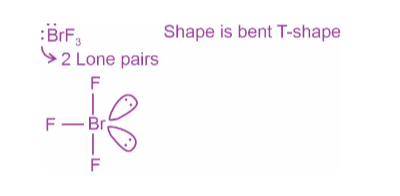11. Aqueous solution of which of the following boron compounds will be strongly basic in nature?

(A) NaBH4

(B) LiBH4

(C) B2H6

(D) Na2B4O7

Sol.

$$\begin{array}{l}Na_2B_4O_7+7H_2O \rightarrow2H_3BO_3+2Na[B(OH)_4]\end{array}$$

Aqueous solution of borax is buffer whose PH ≃ 9

Other compounds are less basic than this.

12. Sulphur dioxide is one of the components of polluted air. SO2 is also a major contributor to acid rain. The correct and complete reaction to represent acid rain caused by SO2 is

(A) 2SO2 + O2 → 2SO3

(B) SO2 + O3 → SO3 + O2

(C) SO2 + H2O2 → H2SO4

(D) 2SO2 + O2 + 2H2O → 2H2SO4

Sol. 2SO2 + O2 + 2H2O → 2H2SO4

Acid rain occurs due to increased concentration of oxides of sulphur and Nitrogen.

13. Which of the following carbocations is most stable?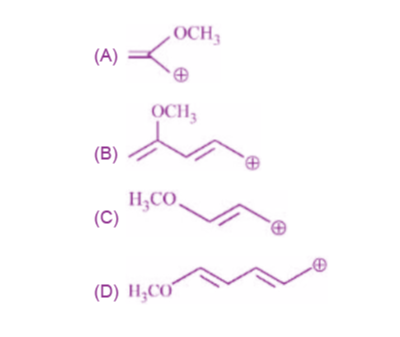Sol.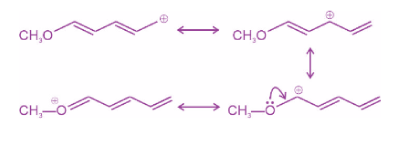14.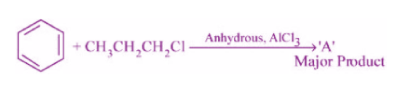The stable carbocation formed in the above reaction is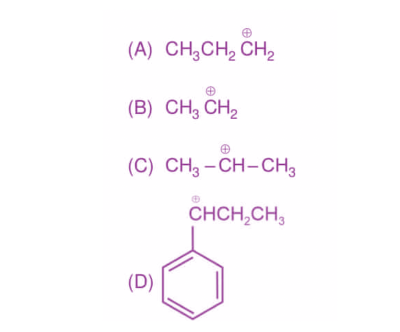Sol. Initially

$$\begin{array}{l}CH_3-CH_2-C\overset{+}{H_2}\end{array}$$
is formed. On rearrangement
$$\begin{array}{l}CH_3-C\overset{+}{H}-CH_3\end{array}$$
stable carbocation is formed.

15. Two isomers (A) and (B) with Molar mass 184 g/mol and elemental composition C, 52.2%; H, 4.9 % and Br 42.9% gave benzoic acid and p-bromobenzoic acid, respectively on oxidation with KMnO4. Isomer ‘A’ is optically active and gives a pale yellow precipitate when warmed with alcoholic AgNO3. Isomers ‘A’ and ‘B’ are, respectively.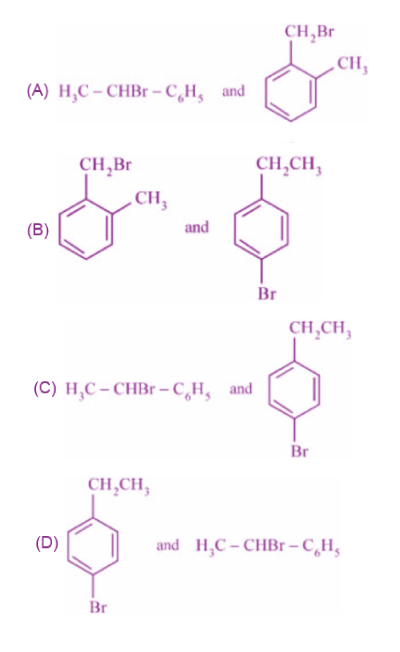Sol.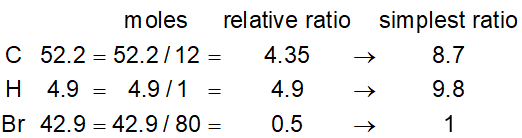C8H9Br

A is optically active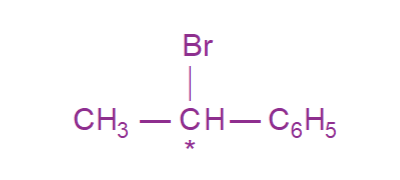B forms para bromo benzoic acid on reaction with KMnO4.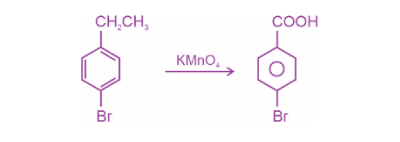16. In Friedel-Crafts alkylation of aniline, one gets

(A) Alkylated product with ortho and para substitution.

(B) Secondary amine after acidic treatment.

(C) An amide product.

(D) Positively charged nitrogen at benzene ring.

Sol.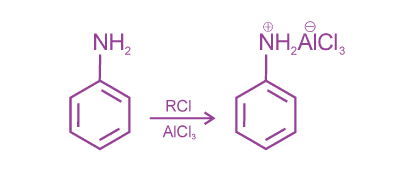17. Given below are two statements : one is labelled as Assertion A and the other is labelled as Reason R.

Assertion A : Dacron is an example of polyester polymer.

Reason R : Dacron is made up of ethylene glycol and terephthalic acid monomers.

In the light of the above statements, choose the most appropriate answer from the options given below.

(A) Both A and R are correct and R is the correct explanation of A.

(B) Both A and R are correct but R is NOT the correct explanation of A.

(C) A is correct but R is not correct.

(D) A is not correct but R is correct.

Sol.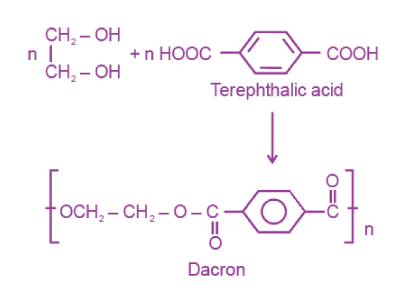18. The structure of protein that is unaffected by heating is

(A) Secondary Structure

(B) Tertiary Structure

(C) Primary Structure

(D) Quaternary Structure

Sol. Primary structure is unaffected by heating

19. The mixture of chloroxylenol and terpineol is an example of

(A) Antiseptic

(B) Pesticide

(C) Disinfectant

(D) Narcotic analgesic

Sol. Mixture of chloroxylenol and terpineol is known as Dettol. It acts as Antiseptic

20. A white precipitate was formed when BaCl2 was added to water extract of an inorganic salt. Further, a gas ‘X’ with characteristic odour was released when the formed white precipitate was dissolved in dilute HCl. The anion present in the inorganic salt is

(A) I

$$\begin{array}{l}(B)\ \textup{SO}_3^{2-}\end{array}$$

(C) S2–

$$\begin{array}{l}(D)\ \textup{NO}_2^{-}\end{array}$$

Sol. Anion is

$$\begin{array}{l}\textup{SO}_3^{2-}\end{array}$$
$$\begin{array}{l}BaSO_3 \xrightarrow{dil. HCl} \underset{\text{X(gas)}}{SO_2} \uparrow \end{array}$$

Gas is released with smell of burning sulphur.

#### SECTION – B

Numerical Value Type Questions: This section contains 10 questions. In Section B, attempt any five questions out of 10. The answer to each question is a NUMERICAL VALUE. For each question, enter the correct numerical value (in decimal notation, truncated/rounded-off to the second decimal place; e.g. 06.25, 07.00, –00.33, –00.30, 30.27, –27.30) using the mouse and the on-screen virtual numeric keypad in the place designated to enter the answer.

1. A box contains 0.90 g of liquid water in equilibrium with water vapour at 27°C. The equilibrium vapour pressure of water at 27°C is 32.0 Torr. When the volume of the box is increased, some of the liquid water evaporates to maintain the equilibrium pressure. If all the liquid water evaporates, then the volume of the box must be ______ litre. [nearest integer]

(Given : R = 0.082 L atm K–1 mol–1]

(Ignore the volume of the liquid water and assume water vapours behave as an ideal gas.)

Sol.

$$\begin{array}{l}\text{H}_2\text{O(l)}\rightleftharpoons\text{H}_2\text{O(g)} \end{array}$$
$$\begin{array}{l}\text{t}=t_{eg} ~~ \dfrac{0.90}{18}-x~~~~~x\end{array}$$

PV = nRT

$$\begin{array}{l}\frac{32}{760}\times V = 0.082 \times (x) \times 300\end{array}$$
$$\begin{array}{l}x=\frac{0.90}{18}\end{array}$$
$$\begin{array}{l}V=0.082 \times \dfrac{0.90}{18} \times \dfrac{300 \times 760}{32}\end{array}$$
$$\begin{array}{l}\approx29.21~L\end{array}$$
$$\begin{array}{l}\approx29~L\end{array}$$

2. 2.2 g of nitrous oxide (N2O) gas is cooled at a constant pressure of 1 atm from 310 K to 270 K causing the compression of the gas from 217.1 mL to 167.75 mL. The change in internal energy of the process, ΔU is ‘–x’ J. The value of ‘x’ is ____. [nearest integer]

(Given : atomic mass of N = 14 g mol–1 and of O = 16 g mol–1

Molar heat capacity of N2O is 100 J K–1 mol–1)

Sol.

$$\begin{array}{l}\Delta \text{T}=-40K\end{array}$$
$$\begin{array}{l}\Delta \text{U}= q+w\end{array}$$
$$\begin{array}{l}=\frac{100 \times 2.2}{44} (-40)-(-49.39) \times10^{-3}\times101.325\end{array}$$

= –200 + 5

= –195 J

x = 195

3. Elevation in boiling point for 1.5 molal solution of glucose in water is 4 K. The depression in freezing point for 4.5 molal solution of glucose in water is 4 K. The ratio of molal elevation constant to molal depression constant (Kb/Kf) is ___.

Sol. ΔTb = i × Kb × m

ΔTf = i × Kf × m i = 1

4 = 1 × Kb × 1.5

––––––––––––––––––––––

4 = 1 × Kf × 4.5

$$\begin{array}{l}\frac{K_b}{K_f} = 3\end{array}$$

4. The cell potential for the given cell at 298 K

Pt | H2 (g, 1 bar) | H+ (aq) || Cu2+ (aq) | Cu(s)

is 0.31 V. The pH of the acidic solution is found to be 3, whereas the concentration of Cu2+ is 10–x M. The value of x is _____.

$$\begin{array}{l}(\text{Given}:\ \mathrm{E}_{\mathrm{Cu}^{2+} / \mathrm{Cu}}^{\Theta}=0.34 \mathrm{~V}\ \text{and}\ \frac{2.303 RT}{F} = 0.06 \text{V})\end{array}$$

Sol.

$$\begin{array}{l}\text{Q}=\frac{\left[\text{H}^{+}\right]^{2}}{\left[\text{Cu}^{+2}\right] \text{pH}_{2}}=\frac{10^{-6}}{\text{C}} \text{pH}_{2}=1\end{array}$$
$$\begin{array}{l}E=\text{E}_{\text {cell }}^{\circ}-\frac{0.06}{\text{n}} \log \text{Q} \end{array}$$
$$\begin{array}{l}0.31=0.34-\frac{0.06}{2} \log \frac{10^{-6}}{\text{C}}\\ \log \frac{10^{-6}}{\text{C}}=1\end{array}$$

C = 10-7 M

x = 7

5. The equation k = (6.5 × 1012s–1)e–26000K/T is followed for the decomposition of compound A. The activation energy for the reaction is ______ kJ mol–1. [nearest integer]

(Given : R = 8.314 J K–1 mol–1]

Sol.

\begin{array}{l}\begin{aligned}&k=A e^{\frac{-E_{a}}{R T}} \\&\frac{E_{a}}{R T}=\frac{26000}{T}\end{aligned}\end{array}

Ea = 26000 × 8.314

= 216164 J

= 216 kJ

6. Spin only magnetic moment of [MnBr6]4– is _____B.M. [round off to the closest integer]

Sol. [MnBr6]–4

x – 6 = –4

x = + 2 Mn = 3d54s2

Mn+2 = 3d54s0

n = 5

$$\begin{array}{l}\mu = \sqrt{n(n+2)}\\ =\sqrt{35} ~\approx \text{6 B.M.} \end{array}$$

7. For the reaction given below:

$$\begin{array}{l}CoCl_3\cdot xNH_3+AgNO_3(aq)\rightarrow\end{array}$$

If two equivalents of AgCl precipitate out, then the value of x will be____.

Sol.

$$\begin{array}{l}[CoCl(NH_3)_5]Cl_2 \xrightarrow{AgNO_3} 2AgCl\downarrow \end{array}$$

x = 5

8. The number of chiral alcohol(s) with molecular formula C4H10O is ____.

Sol.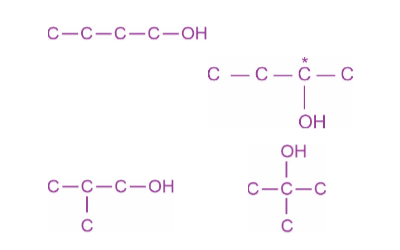9. In the given reaction,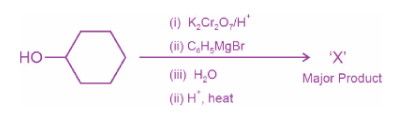the number of sp2 hybridised carbon(s) in compound ‘X’ is _____.

Sol.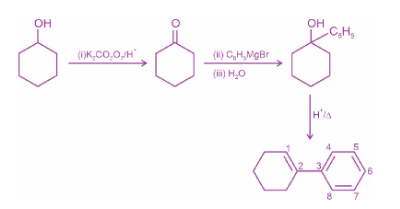10. In the given reaction,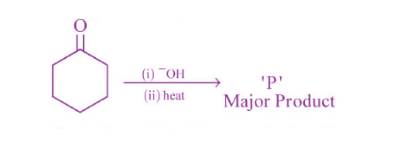The number of π electrons present in the product ‘P’ is_____.

Sol.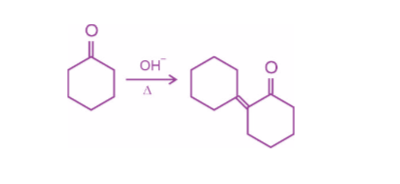Total 4π electrons are there. Reaction is aldol condensation.

### Download PDF of JEE Main 2022 June 29 Shift 2 Chemistry Paper & Solutions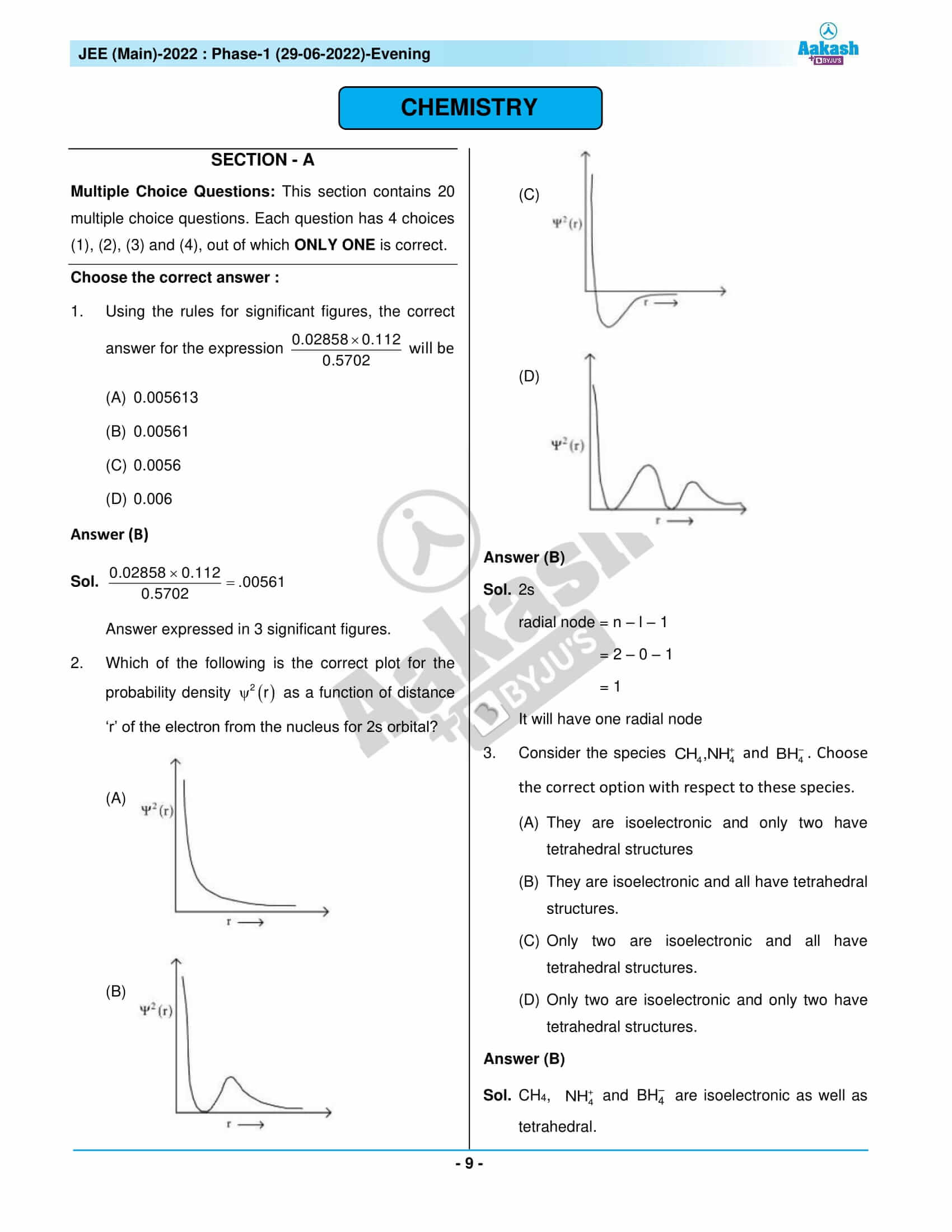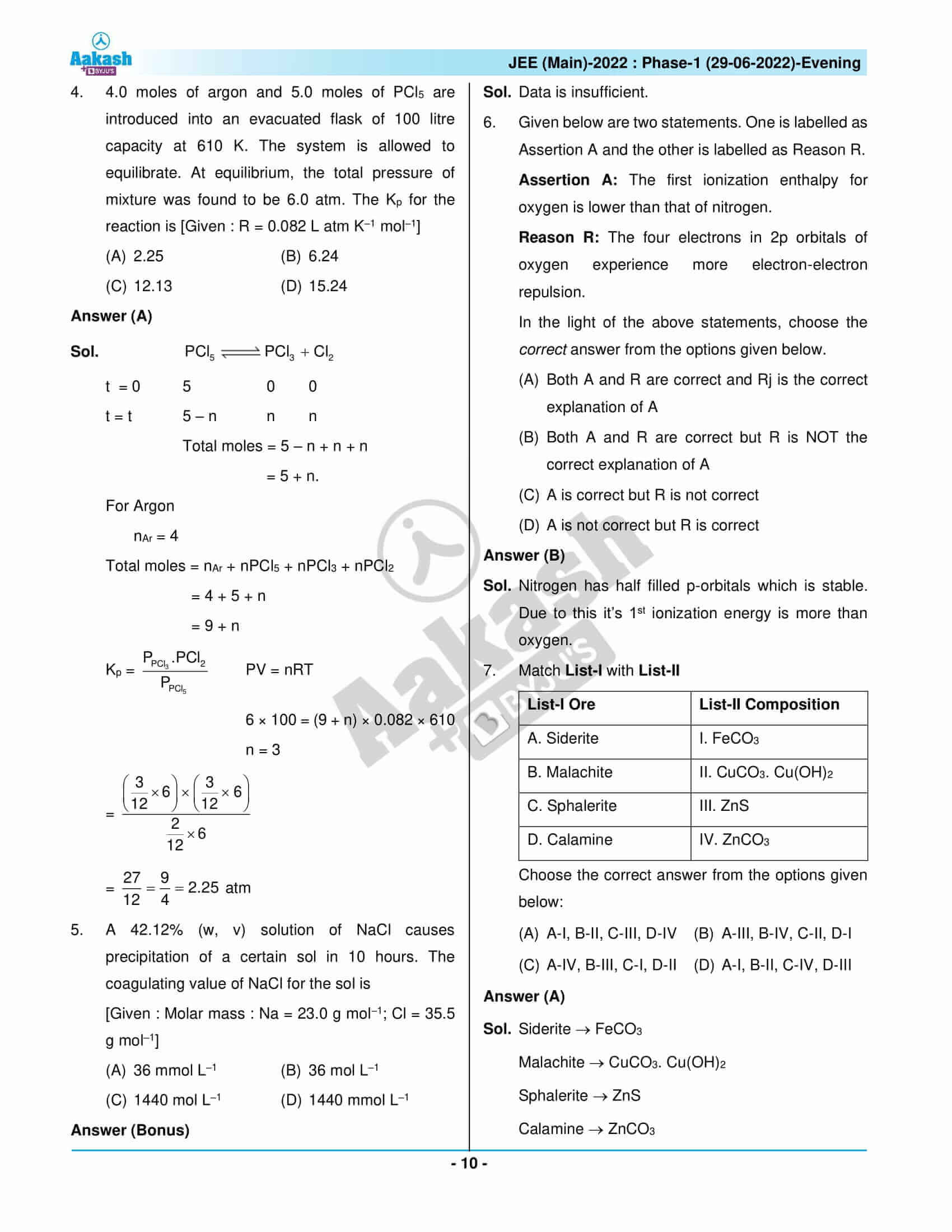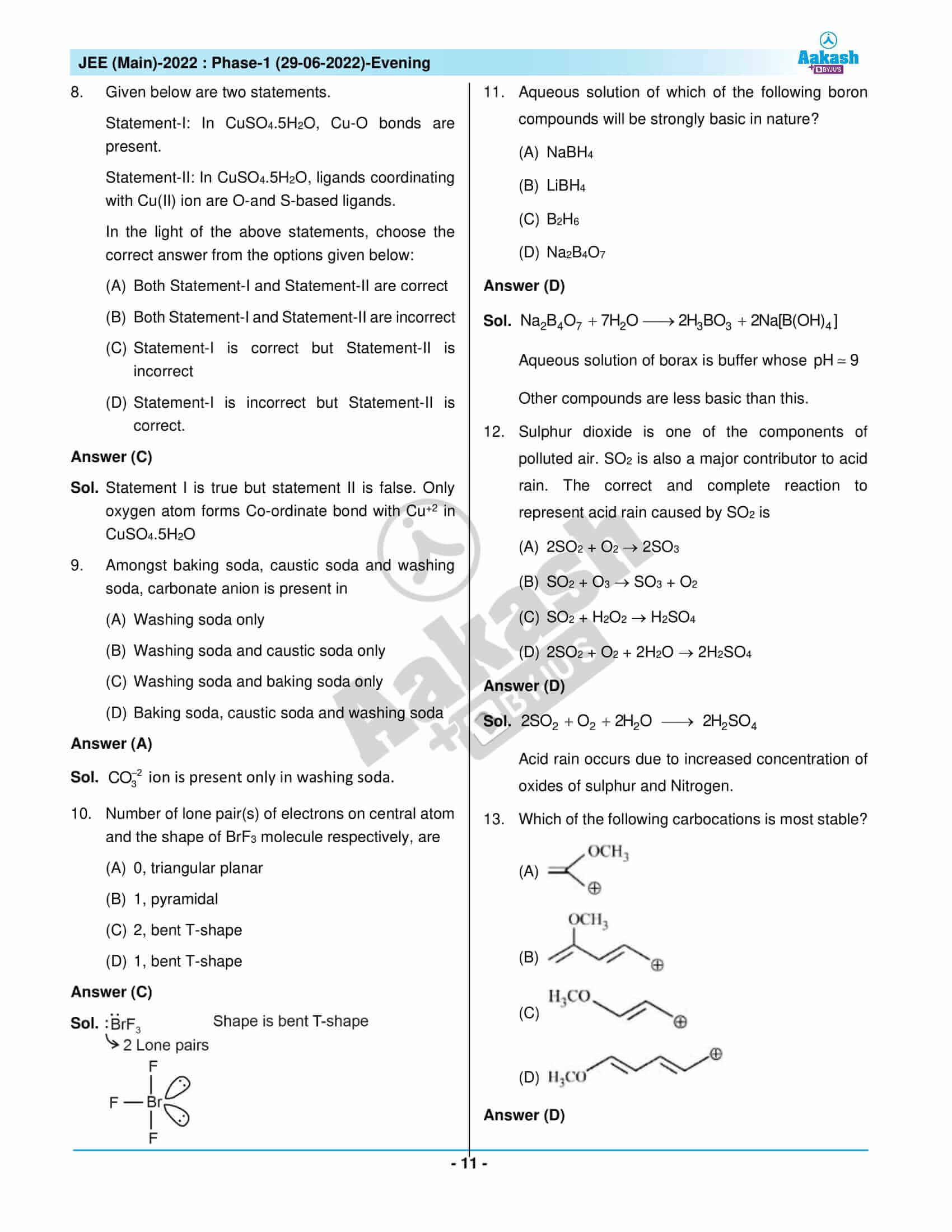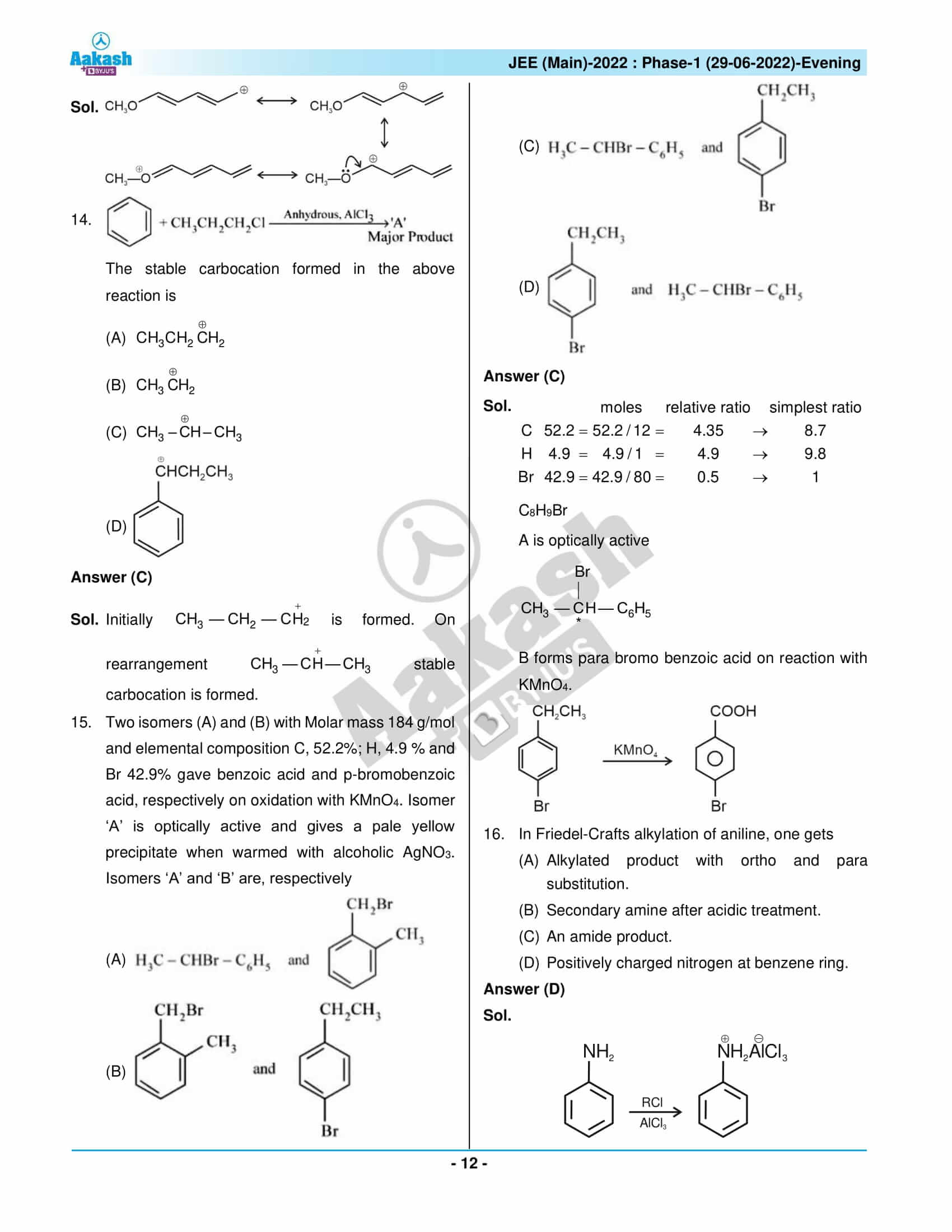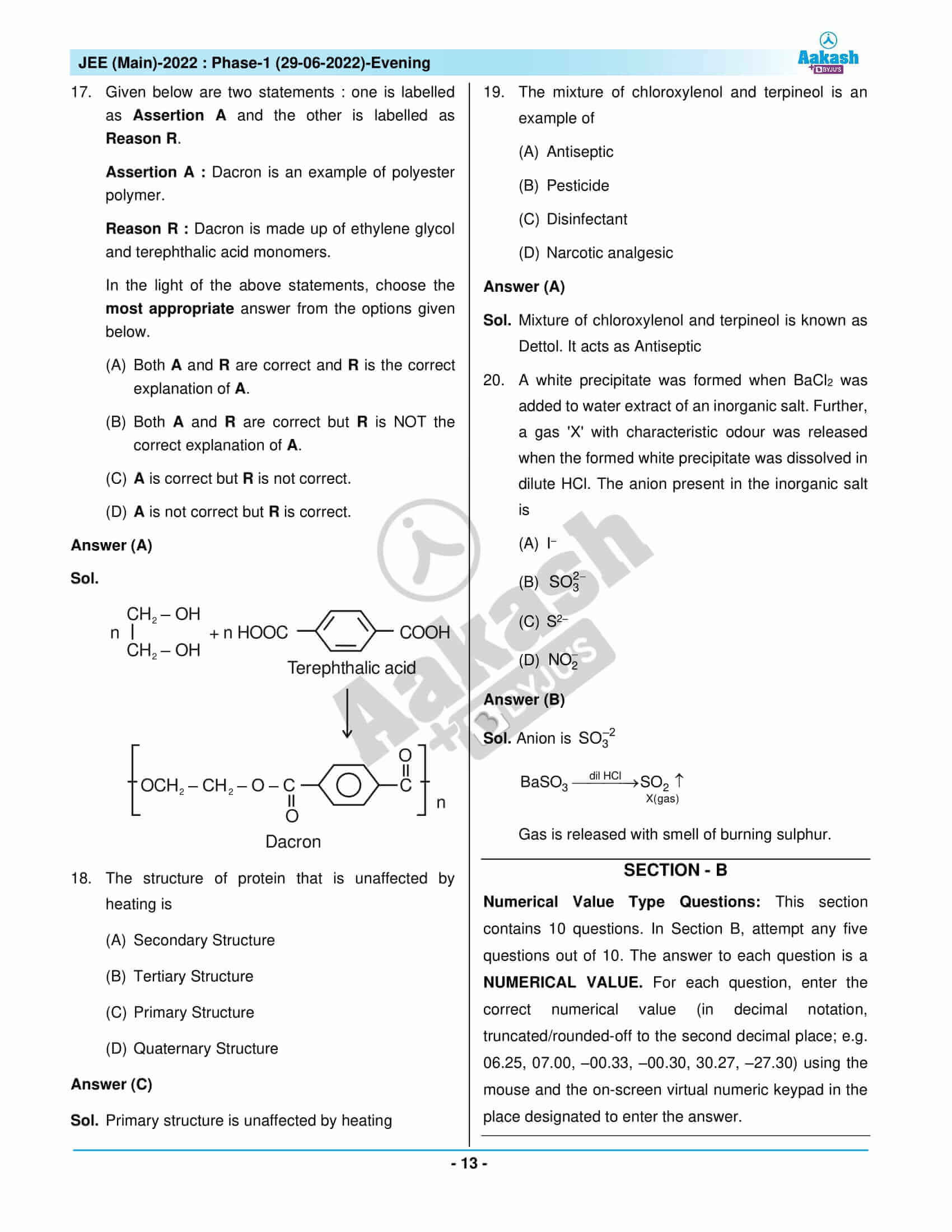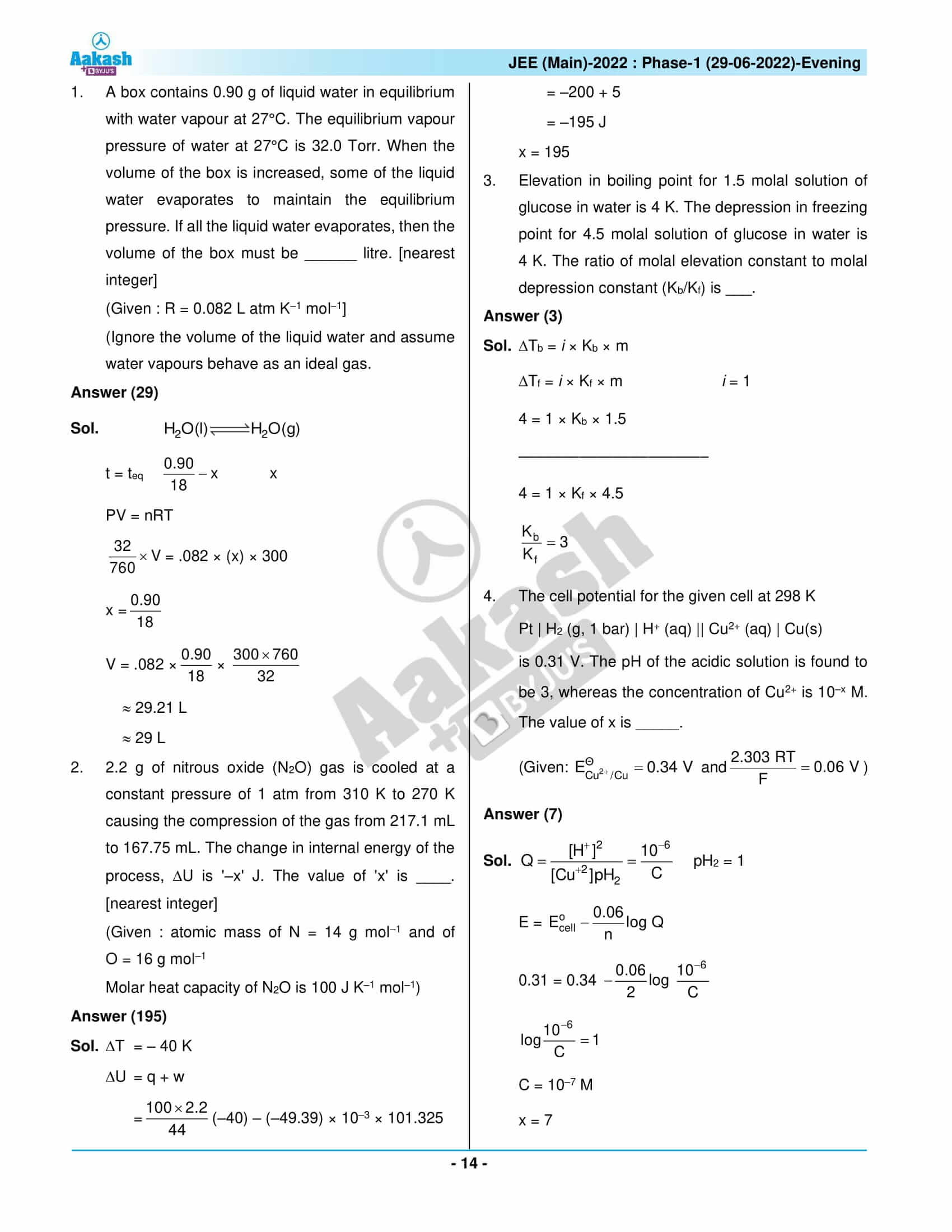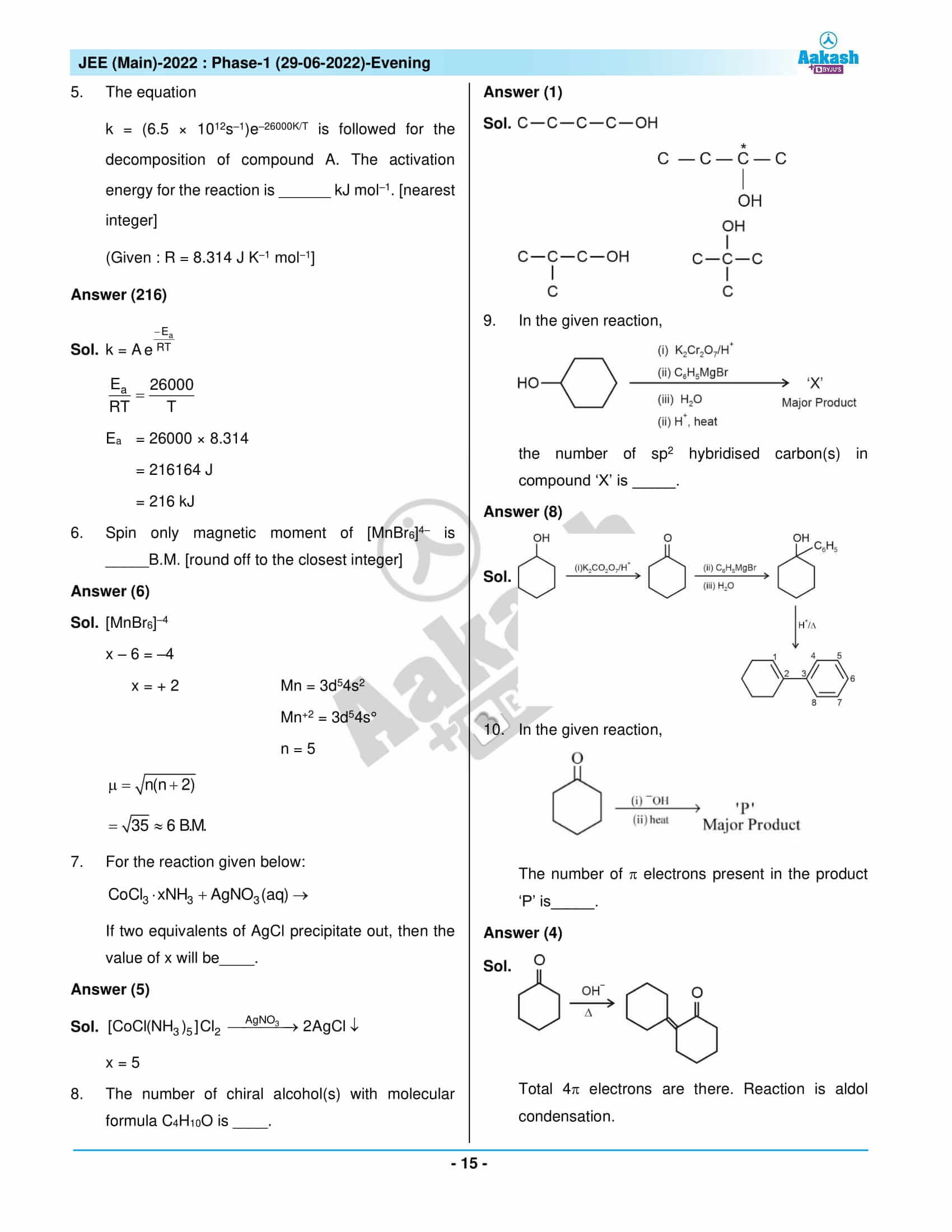## JEE Main 2022 Paper with Solutions – 29 June Shift 2

### JEE Main 2022 June 29 Shift 2 Question Paper – Physics Solutions### JEE Main 2022 June 29 Shift 2 Question Paper – Chemistry Solutions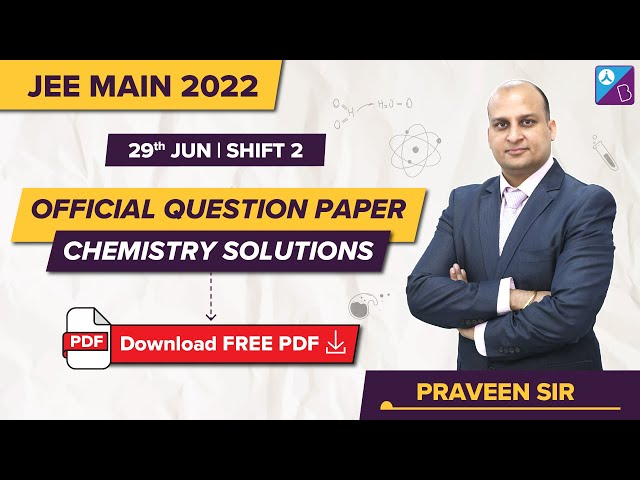### JEE Main 2022 June 29 Shift 2 Question Paper – Maths Solutions## Frequently Asked Questions – FAQs

Q1

### Was the Chemistry section of the JEE Main 2022 June 29 – Shift 2 Question Paper difficult?

Questions in Chemistry ranged from moderate to difficult. 9 medium, 13 easy, and 1 difficult questions were in the chemistry section based on the analysis of the 23 memory-based questions.

Q2

### List a few topics from which Chemistry questions were asked in the JEE Main 2022 June 29 afternoon shift question paper.

A few Chemistry topics from which questions were asked in JEE Main 2022 June 29 afternoon shift question paper are
Magnetic properties
Compounds of Sodium and Potassium
Mole Concept and Molar Masses
Soil Pollution
Antimicrobials
Classification of Polymerisation Reaction
Atmospheric Pollution
Pressure-Volume Work

Q3

### List a few Chemistry chapters included from the Class 11 syllabus in JEE Main 2022 June 29 afternoon shift question paper.

The chapters included are:
The S-Block Elements
Some Basic Concepts of Chemistry
Environmental Chemistry
Chemical Bonding and Molecular Structure
Thermodynamics
Hydrocarbons
Organic Chemistry Some Basic Principles and Techniques
Structure of Atom
States of Matter

Q4

### What was the class-wise question distribution of Chemistry questions in JEE Main 2022 June 29 Shift 2 question paper?

As per our expert faculties, the questions from the Class 12th syllabus and the Class 11th syllabus had an approximately identical proportion. Of the 23 Chemistry memory-based questions, 10 questions are from Class 11 and 13 questions are from Class 12.

Q5

### What was the rating given to the difficulty level of the Chemistry questions in JEE Main 2022 June 29 Shift 2 question paper?

The rating given by the expert faculty to the Physics question’s difficulty level is 1.43 on a scale of 1 to 3.